## Cubic Spline interpolation in C++

#### Features

• interpolates grid points (xi, yi) with cubic C2 splines or C1 Hermite splines or C1 monotonic splines
• light weight, simple to use, no dependencies
• efficient: O(N) to generate spline, O(log(N)) to evaluate the spline at a point

It is implemented as a single header file:

#### Usage

#include <cstdio>
#include <vector>
#include "spline.h"

int main(int, char**) {
std::vector<double> X = {0.1, 0.4, 1.2, 1.8, 2.0}; // must be increasing
std::vector<double> Y = {0.1, 0.7, 0.6, 1.1, 0.9};

tk::spline s(X,Y);
double x=1.5, y=s(x), deriv=s.deriv(1,x);

printf("spline at %f is %f with derivative %f\n", x, y, deriv);
}

Compile:
$g++ -Wall -std=c++11 demo.cpp -o demo$ ./demo
spline at 1.500000 is 0.915345 with derivative 1.223601


#### Spline types

Different spline types can be specified in the constructor or via the set_points() method.
cubic C2 spline cubic C1 Hermite spline
• f(xi) = yi
• twice continuously differentiable
• f(xi) = yi
• once continuously differentiable
• f'(xi) = finite_difference(i-1, i, i+1) = (yi+1-yi-1)/(xi+1-xi-1) on a uniform x-grid
    tk::spline s(X,Y,tk::spline::cspline); // or tk::spline s(X,Y);
// alternatively
tk::spline s2;
s2.set_points(X,Y,tk::spline::cspline); // or s2.set_points(X,Y);

    tk::spline s(X,Y,tk::spline::cspline_hermite);
// alternatively
tk::spline s2;
s2.set_points(X,Y,tk::spline::cspline_hermite);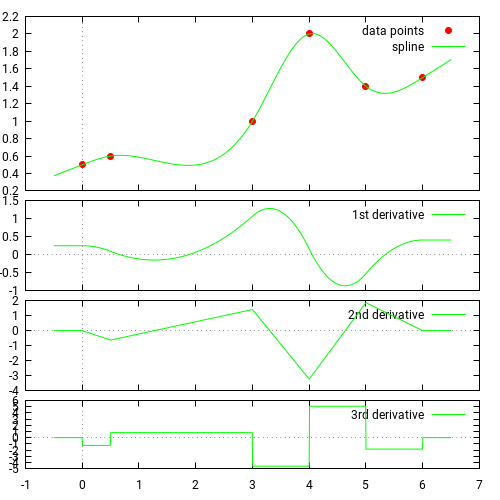$./plot.sh -t cspline -x 0 0.5 3 4 5 6 -y 0.5 0.6 1 2 1.4 1.5$ ./plot.sh -t hermite -x 0 0.5 3 4 5 6 -y 0.5 0.6 1 2 1.4 1.5

changing a grid point has global implications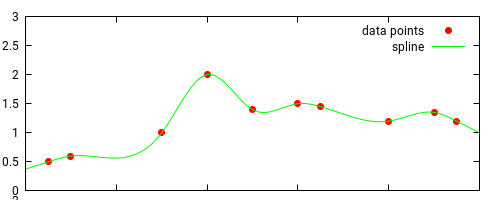changing a grid point only impacts 2 segments to the left and right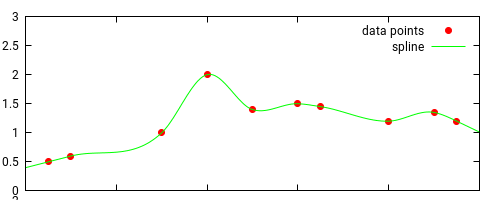Source:

#### Monotonic splines

If input data is monotonic and the resulting spline is not monotonic, it can be enforced via the make_monotonic() method. Internally, this is achieved by reducing the slope on grid points adjacent to non-monotonic segments (this breaks C2 and the resulting spline is only C1).
cubic C2 spline cubic monotonic spline
    tk::spline s(X,Y,tk::spline::cspline); // or tk::spline s(X,Y);
// alternatively
tk::spline s2;
s2.set_points(X,Y,tk::spline::cspline); // or s2.set_points(X,Y);

    tk::spline s(X,Y,tk::spline::cspline,true);
// alternatively
tk::spline s2;
s2.set_points(X,Y,tk::spline::cspline);
s2.make_monotonic();$./plot.sh -t cspline -x 0 0.5 3 4 5 6 -y 0.5 0.6 1 2 2.1 2.5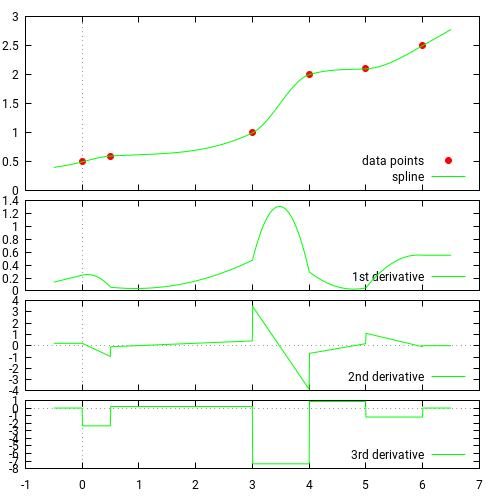$ ./plot.sh -t cspline -x 0 0.5 3 4 5 6 -y 0.5 0.6 1 2 2.1 2.5 -m

Source:

#### Boundary conditions

Left and right boundary conditions can be set via the constructor or the set_boundary() method (must be called before the set_points() method). Periodic boundary conditions are currently not implemented.
first derivatives second derivatives
e.g. set left and right slope to zero:
    tk::spline sp(X,Y,type,false,
tk::spline::first_deriv,0.0,
tk::spline::first_deriv,0.0);
// alternatively
tk::spline s;
s.set_boundary(tk::spline::first_deriv, 0.0,
tk::spline::first_deriv, 0.0);
s.set_points(X,Y,type);

e.g. set left and right curvature to zero:
    tk::spline sp(X,Y,type,false,
tk::spline::second_deriv,0.0,
tk::spline::second_deriv,0.0);
// alternatively
tk::spline s;
s.set_boundary(tk::spline::second_deriv, 0.0,
tk::spline::second_deriv, 0.0);
s.set_points(X,Y,type);


#### Mean preserving splines (average preserving, area preserving, mass preserving)

Sometimes one is given averages and needs to plot a smooth curve which has equal averages, e.g. for histograms. Then the spline does not interpolate points but has to satisfy the integral condition:
• $\frac{1}{x_{i+1}-x_i} \int_{x_i}^{x_{i+1}} f(x) \text{d}x = \text{avg}_i$
• $f\in\text{C}^1$, or at least $f\in\text{C}^0$
• sometimes $f(x)\geq 0$ is required
Luckily, this problem can be transformed so that any interpolating spline can be used here. Define the integral function $F(x) = \int_{x_0}^x f(t) \text{d}t$, then the above conditions are equivalent to
• $F(x_{i+1}) = F(x_i) + \text{avg}_i \, (x_{i+1}-x_i)$
• $F\in\text{C}^2$, or at least $F\in\text{C}^1$
• sometimes $F(x)$ is required to be monotonic
In code this means:
    Y=0.0;
for(int i=1; i<n; i++)
Y[i] = Y[i-1] + avg[i-1]*(X[i]-X[i-1]);
tk::spline s(X,Y);
double interpol = s.deriv(1,x);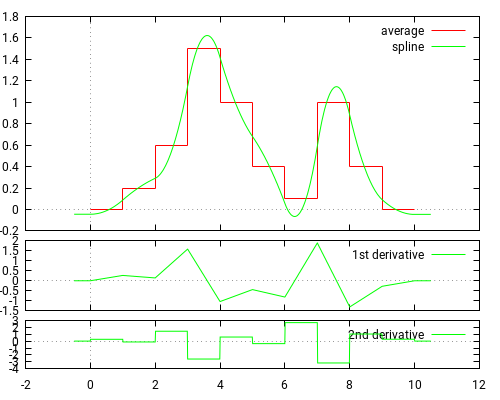$./plot_avg_preserv.sh -y 0 0.2 0.6 1.5 1 0.4 0.1 1 0.4 0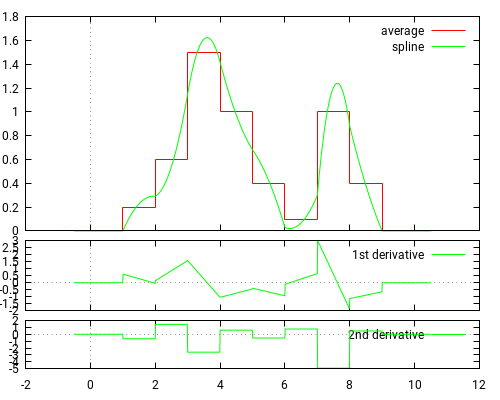$ ./plot_avg_preserv.sh -y 0 0.2 0.6 1.5 1 0.4 0.1 1 0.4 0 -m

Source:

#### Parametric splines and closed splines

To connect arbitrary points in a 2D plane (or any n-D space) we can simply introduce a "time" variable and treat every coordinate as a function of time, i.e. every coordinate is interpolated via a separate spline:
    // time proportional to distance, i.e. traverse the curve at constant speed
T=0.0;
for(size_t i=1; i<T.size(); i++)
T[i] = T[i-1] + sqrt( (X[i]-X[i-1])*(X[i]-X[i-1]) + (Y[i]-Y[i-1])*(Y[i]-Y[i-1]) );
// setup splines for x and y coordinate
tk::spline sx(T,X), sy(T,Y);
...
double x = sx(t);
double y = sy(t);

parametric spline parametric closed spline
• using natural boundary conditions, i.e. f''(a)=f''(b)=0
• first and last point identical, i.e. x0=xn, y0=yn
• would need to use periodic boundary conditions, i.e. f'(a)=f'(b)
• as a hack can also use natural boundary conditions and traverse the closed curve a few times before drawing (done here)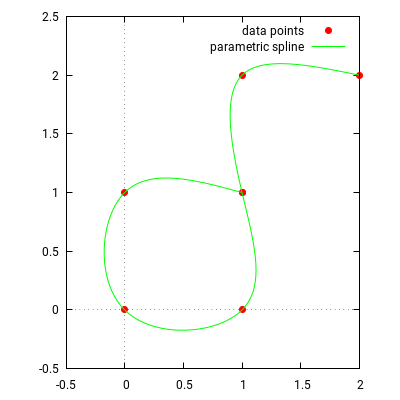$./plot_parametric.sh -x 1 0 0 1 1 1 2 -y 1 1 0 0 1 2 2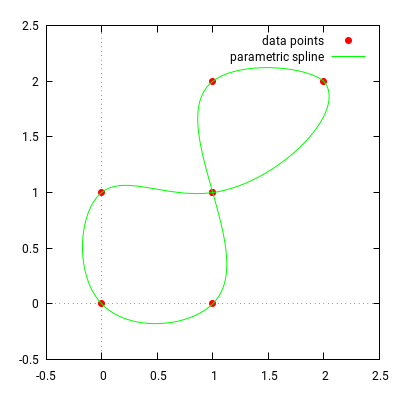$ ./plot_parametric.sh -x 1 0 0 1 1 1 2 -y 1 1 0 0 1 2 2 -c

Source:

#### Comparison with ALGLIB

The result of this library is compared against ALGLIB. Interpolation results are identical but extrapolation differs, as tk::spline is designed to extrapolate linearly.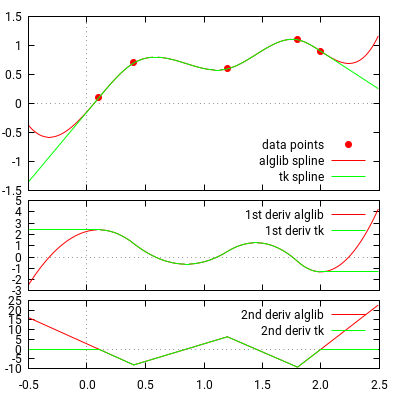Source:

#### Benchmark

A simple benchmark shows that performance is comparable with ALGLIB. The following operations are measured:
• Spline creation: calculate the coefficients of a spline given input vectors X and Y,
• Random access: evaluate spline(x), i.e. y-value of the spline at a random point x,
• Grid transform: given vectors X1,Y1, and a new vector X2, then we want to calculate the corresponding Y2 vector, i.e. Y2[i]=spline(X2[i]). Alglib outperforms here, because this operation can be implemented more efficiently (TODO list).
The output "cycl" is the number of cpu cycles required for a single operation.
$./bench usage: ./bench <cpu mhz> <spline size> <loops in thousand>$ ./bench 2666 10 10000	    # splines based on 10 grid points, 10million loops
tk                      alglib
random access:   loops=1e+07,   0.202s (  54 cycl)      0.407s ( 108 cycl)
spline creation: loops=1e+06,   2.067s (5511 cycl)      3.391s (9039 cycl)
grid transform:  loops=1e+06,   2.437s (6497 cycl)      3.132s (8351 cycl)

accuracy: max difference = 5.55e-16, l2-norm difference = 2.46e-20

$./bench 2666 10000 10000 # splines based on 10000 grid points, 10million loops tk alglib random access: loops=1e+07, 1.390s ( 371 cycl) 1.534s ( 409 cycl) spline creation: loops=1e+03, 1.674s (4.5e+06 cycl) 1.793s (4.8e+06 cycl) grid transform: loops=1e+03, 2.212s (5.9e+06 cycl) 1.424s (3.8e+06 cycl) accuracy: max difference = 4.41e-13, l2-norm difference = 7.85e-19  Source: Compile as follows where the alglib source and compiled lib needs to be in the appropriate directory: $ g++ -Wall -O2 -I../alglib/src -c bench.cpp -o bench.o
$g++ bench.o -o bench -L../alglib/ -lalglib  ### Maths Below is just an outline. For more details see spline.pdf. You need to enable JavaScript to see the maths symbols on this page.$ \newcommand{\R}{\mathbb{R}} \newcommand{\Co}{\mathrm{C}} $Input: a set of ordered$x, y$coordinates \begin{equation*} \{(x_1,y_1),\dots,(x_n,y_n)\},\quad \text{with } x_1 < x_2 < \dots < x_n. \end{equation*} Output: a piecewise cubic function$f_i:[x_i,x_{i+1}]\to\R$, \begin{equation} f_i(x) = a_i + b_i (x-x_i) + c_i (x-x_i)^2 + d_i (x-x_i)^3. \end{equation} Define the space between$x$-points by$h_i:=x_{i+1}-x_i$. #### Cubic C2 splines Defined by •$f(x_i) = y_i$, i.e.$f$interpolates all points, •$f\in\Co^2$, i.e.$fis twice continuously differentiable. In detail this means: \begin{equation*} \begin{aligned} \text{interpolates points: } f_i(x_i) & = y_i: & a_i & = y_i,\\ \text{continuous } f\in\Co^0 \text{: } f_i(x_{i+1}) & =y_{i+1}: & d_i h_i^3 + c_i h_i^2 + b_i h_i & = y_{i+1}-y_i,\\ \text{differentiable }f\in\Co^1 \text{: } f'_{i-1}(x_i) & = f'_i(x_i): & 3 d_{i-1} h_{i-1}^2 + 2 c_{i-1} h_{i-1} + b_{i-1} & = b_i,\\ \text{twice differentiable }f\in\Co^2 \text{: } f''_i(x_{i+1}) & = f''_{i+1}(x_{i+1}): & 6 d_i h_i + 2 c_i & = 2 c_{i+1},\\ \end{aligned} \end{equation*} This can be solved to obtain a sparse linear equation system inc_i: \begin{equation} \label{eq:cubic_c2_spline_coeffs} \boxed{ \begin{aligned} \frac{1}{3} h_{i-1} c_{i-1} + \frac{2}{3} (h_{i-1}+h_i) c_i + \frac{1}{3} h_i c_{i+1} & = \frac{y_{i+1}-y_i}{h_i} - \frac{y_i-y_{i-1}}{h_{i-1}}, & i\in\{2,\dots,n-1\},\\ d_i & =\frac{c_{i+1}-c_i}{3 h_i}, & i\in\{1,\dots,n-1\},\\ b_i & = \frac{y_{i+1}-y_i}{h_i} - \frac{1}{3} (2 c_i + c_{i+1}) h_i, & i\in\{1,\dots,n-1\}. \end{aligned} } \end{equation} #### Hermite C1 splines Defined by •f(x_i) = y_i$, i.e.$f$interpolates all points, •$f\in\Co^1$, i.e.$f$is continuously differentiable, •$f'(x_i) = \delta_i, i.e. derivatives are specified at each inner point, e.g. by three point finite differences \begin{equation*} \delta_i=-\frac{h_i}{h_{i-1}(h_{i-1} + h_i)} y_{i-1} + \frac{h_i-h_{i-1}}{h_{i-1} h_i} y_i + \frac{h_{i-1}}{h_i(h_{i-1} + h_i)} y_{i+1}. \end{equation*} In detail this means: \begin{equation*} \begin{aligned} \text{interpolates points: } f_i(x_i) & = y_i: & a_i & = y_i,\\ \text{prescribed derivative: } f'_i(x_i) & = \delta_i: & b_i & = \delta_i,\\ \text{continuous } f\in\Co^0 \text{: } f_i(x_{i+1}) & =y_{i+1}: & d_i h_i^3 + c_i h_i^2 + b_i h_i & = y_{i+1}-y_i,\\ \text{differentiable }f\in\Co^1 \text{: } f'_i(x_{i+1}) & =\delta_{i+1}: & 3 d_i h_i^2 + 2 c_i h_i & = \delta_{i+1}-\delta_i,\\ \end{aligned} \end{equation*} which can be solved to obtain \begin{equation} \label{eq:hermite_spline_coeffs} \boxed{ \begin{aligned} a_i & = y_i, \\ b_i & = \delta_i, \\ c_i & = -\frac{2 b_i+b_{i+1}}{h_i}+3\frac{a_{i+1}-a_i}{h_i^2} & = -\frac{2\delta_i+\delta_{i+1}}{h_i} + 3 \frac{y_{i+1}-y_i}{h_i^2}, \quad i\in\{1,\dots,n-1\},\\ d_i & = -\frac{2 c_i}{3 h_i}+\frac{b_{i+1}-b_i}{3 h_i^2} & = \frac{\delta_i+\delta_{i+1}}{h_i^2}-2\frac{y_{i+1}-y_i}{h_i^3}, \quad i\in\{1,\dots,n-1\}. \end{aligned} } \end{equation} #### Monotonic C1 splines A sufficient (but not necessary) condition for monotinicity is \begin{equation} \sqrt{b_i^2+b_{i+1}^2} \leq 3 \frac{y_{i+1}-y_i}{h_i}. \end{equation} This can always be achieved by re-scalingb_i$and then re-calculating$c_i$and$d_i\$ based on \eqref{eq:hermite_spline_coeffs}. See also Monotone piecewise cubic interpolation by Fritsch and Carlson.

Math symbols are rendered by MathJax which requires JavaScript.